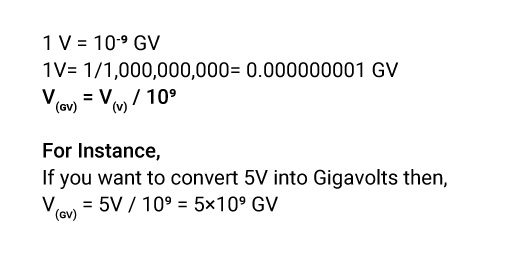## About Our V to GV Converter

“Although playing with numbers is challenging, it is great therapy for brain’s health.”

These days, you don’t need to go through lengthy calculations or mug-up formulas since we proudly introduce a helpful converter that will provide accurate calculations in a flash. If you want to switch Volts to Gigavolts or looking for any metric conversion, you will find details of each measurement in a click.

### How to Convert Volts to Gigavolts?

Well, too many zeroes often confuse people. Let us get straight. To convert volts to Gigavolts, divide the Voltage by the conversion ratio. One Gigavolts is equal to the Voltage divided by 1,000,000,000. One volt equals one-billionth of a GV.

### The Volts to Gigavolts Conversion Formula.

Here is the direct volts to Gigavolts conversion formula for a simple calculation.You may find it a bit puzzling. If the formula confuses you, our converter will help you. Convert and get the answer with just a click using the volts to Gigavolts calculator.

### How to Use Our Volts to Gigavolts Converter?

Ease your worries by using our volts to Gigavolts converter.

• Step2: Present the value of Voltage.
• Step3: Click “convert” to convert volts to Gigavolts.

You can enter any value you want to switch to Gigavolts. Make sure that you click on the covert every time you enter a new value to get the result.

### How Many Volts Are in a Gigavolts?

1 GV = 109 V

1 GV= 1000000000 V

One Gigavolt is equal to one billion volts.

### What is the Difference Between Volts & Gigavolts?

• Volt is the SI unit of Voltage, i.e., the electrical potential difference between 2 points of a conductor. The symbol of volts is V. One volt is the one-billionth of a GV.
• Gigavolts is a multiple unit of Voltage. It is typically used in large quantities. The symbol of Gigavolts is GV. One Gigavolts equals one billion volts.

### Examples of Converting Volts to Gigavolts.

The examples below will help you convert volts to Gigavolts more easily.

V(GV) = 4V / 109 = 4×109 GV

V(GV) = 6V / 109 = 6×109 GV

V(GV) = 8V / 109 = 8×109 GV

V(GV) = 9V / 109 = 9×109 GV

### Volt to Gigavolts Conversion Table.

Give a sight at Instant volt to Gigavolts conversion table.

Volts Gigavolts
1V 10-9 GV
10V 10-8 GV
100V 10-7 GV
1,000V 10-6 GV
10,000V 10-5 GV
1,00,000 V 0.0001 GV